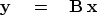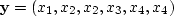Next: Looping over input space Up: NORMAL MOVEOUT AND OTHER Previous: Nearest-neighbor interpolation

## A family of nearest-neighbor interpolations

Let an integer k range along a survey line, and let data values xk be packed into a vector.(Each data point xk could also be a seismogram.) We plan to resample the data more densely, say from 4 to 6 points. For illustration, I follow a crude nearest-neighbor interpolation

scheme by sprinkling ones along the diagonal of a rectangular matrix that is(10)
where(11)
The interpolated data is simply.The matrix multiplication (11) would not be done in practice. Instead there would be a loop running over the space of the outputsthat picked up values from the input.Next: Looping over input space Up: NORMAL MOVEOUT AND OTHER Previous: Nearest-neighbor interpolation
Stanford Exploration Project
10/21/1998